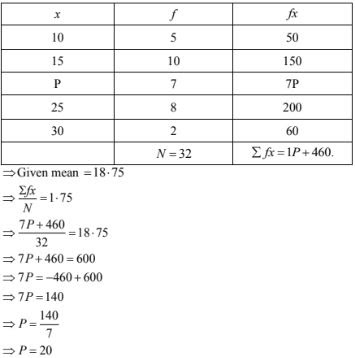# RD Sharma Solutions Chapter 7 Statistics Exercise 7.1 Class 10 Maths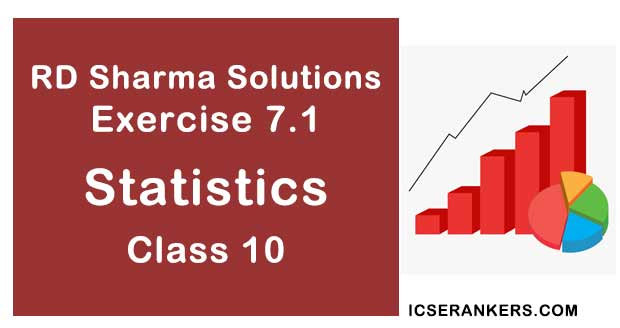Chapter Name RD Sharma Chapter 7 Statistics Book Name RD Sharma Mathematics for Class 10 Other Exercises Exercise 7.2Exercise 7.3Exercise 7.4Exercise 7.5Exercise 7.6 Related Study NCERT Solutions for Class 10 Maths

### Exercise 7.1 Solutions

1 .Calculate the mean for the following distribution :
 x : 5 6 7 8 9 f : 4 8 14 11 3

Solution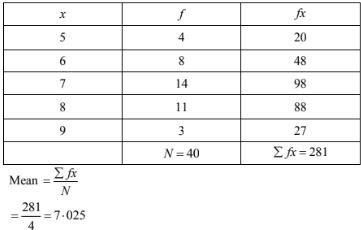2 .Find the mean of the following data :
 x : 19 21 23 25 27 29 31 f: 13 15 16 18 16 15 13

Solution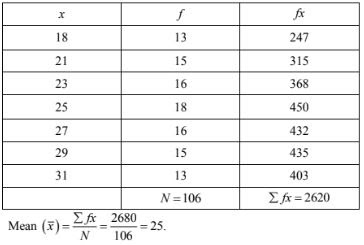3 .If the mean of the following data is 20.6. Find the value of p.
 x 10 15 P 25 35 f 3 10 25 7 5
Solution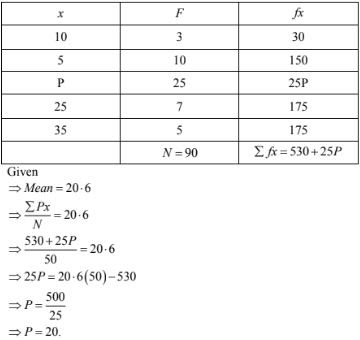4 .If the mean of the following data is 15, find p.
 x 5 10 15 20 25 f 6 P 6 10 5
Solution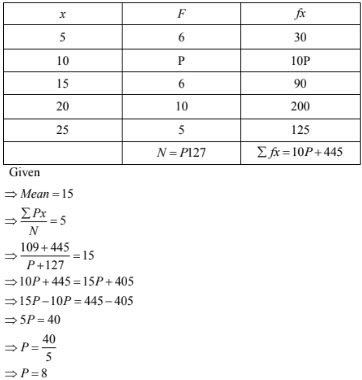5. Find the value of p for the following distribution whose mean is 16.6
 x 8 12 15 P 20 25 30 f 12 16 20 24 16 8 4
Solution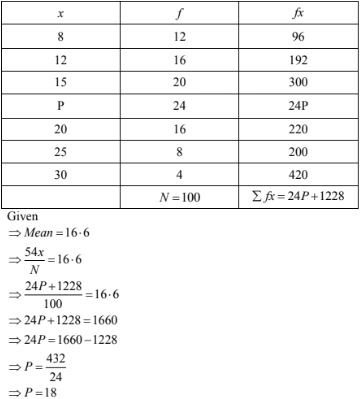6. Find the missing value of p for the following distribution whose mean is 12.58
 x 5 8 10 12 P 20 25 f 2 5 8 22 7 4 2
Solution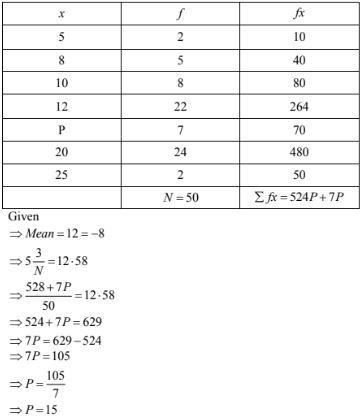7. Find the missing frequency (P) for the following distribution whose mean is 7.68
 x 3 5 7 9 11 13 f 6 8 15 P 8 4
Solution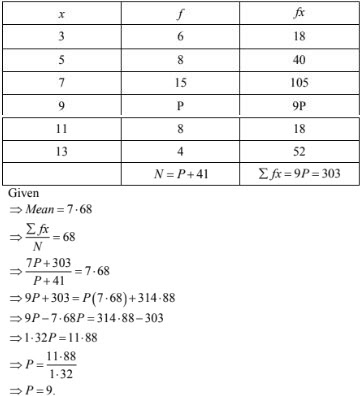8. Find the value of p, if the mean of the following distribution is 20.
 x 15 17 19 20+p 23 f 2 3 4 5p 6
Solution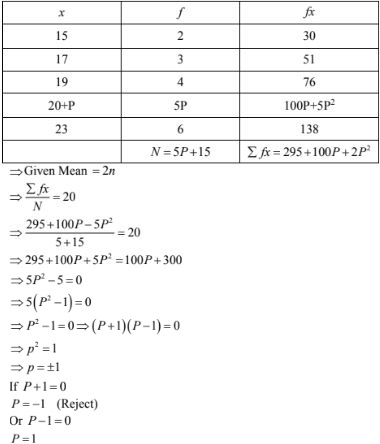9. The following table gives the number of boys of a particular age in a class of 40 students.
Calculate the mean age of the students.
 Age(in years) 15 16 17 18 19 20 No. of students: 3 8 10 10 5 4
Solution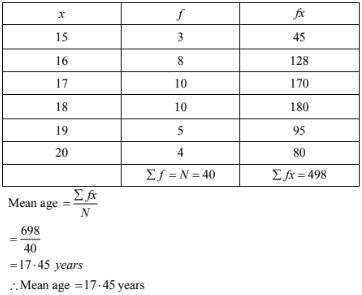10. Candidates of four schools appear in a mathematics test. The data were as follows:
 Schools No. of Candidates Average Score I 60 75 II 48 80 III NA 55 IV 40 50

If the average score of the candidates of all the four schools is 66, find the number of candidates that appeared from school III.

Solution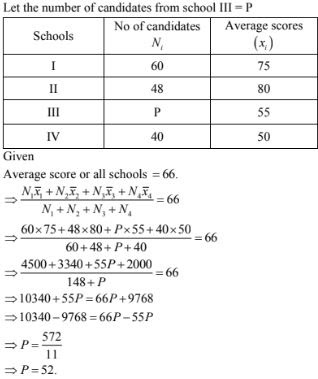11. Five coins were simultaneously tossed 1000 times and at each toss the number of heads were observed. The number of tosses during which 0, 1, 2, 3, 4 and 5 heads were obtained are shown in the table below. Find the mean number of heads per toss.
 No. of heads per toss No. of tosses 0 38 1 144 2 342 3 287 4 164 5 25 Total 1000
Solution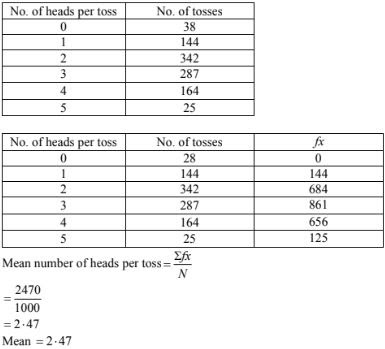12. Find the missing frequencies in the following frequency distribution if it is known that the mean of the distribution is 50.
 X 10 30 50 70 90 F 17 F1 32 F2 19
Solution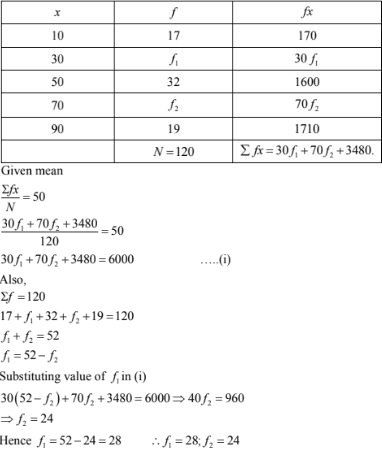13. The arithmetic mean of the following data is 14. Find the value of k
 Xi 5 10 15 20 25 Fi 7 K 8 4 5
Solution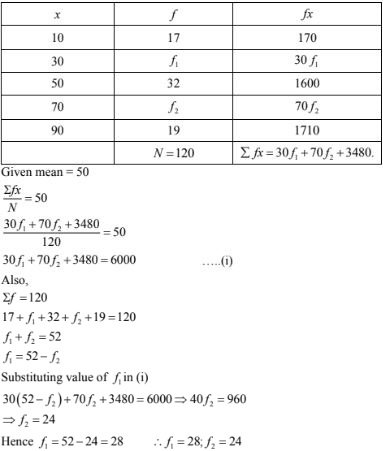14. The arithmetic mean of the following data is 25, find the value of k.
 Xi 5 15 25 35 45 Fi 3 K 3 6 2
Solution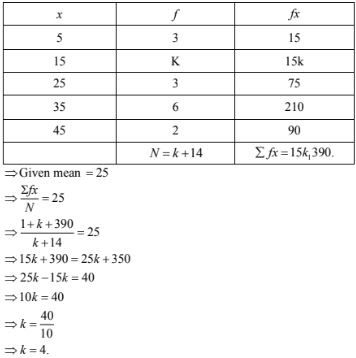15. If the mean of the following data is 18.75. Find the value of p.

 Xi 10 15 P 25 30 Fi 5 10 7 8 2
Solution